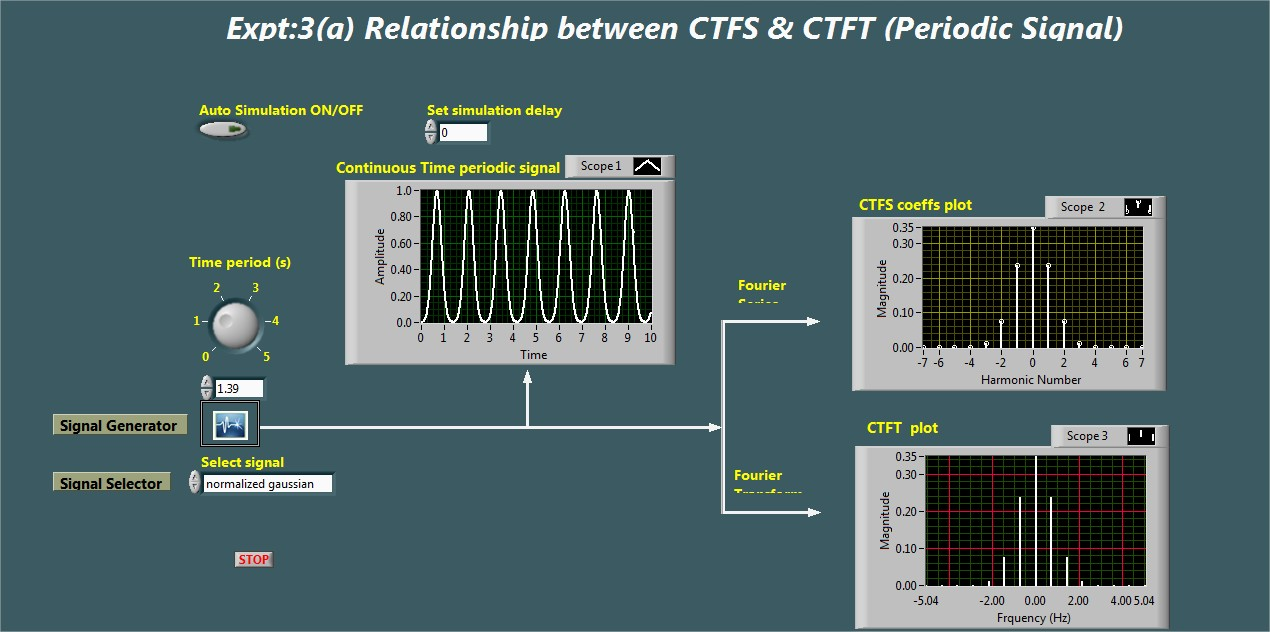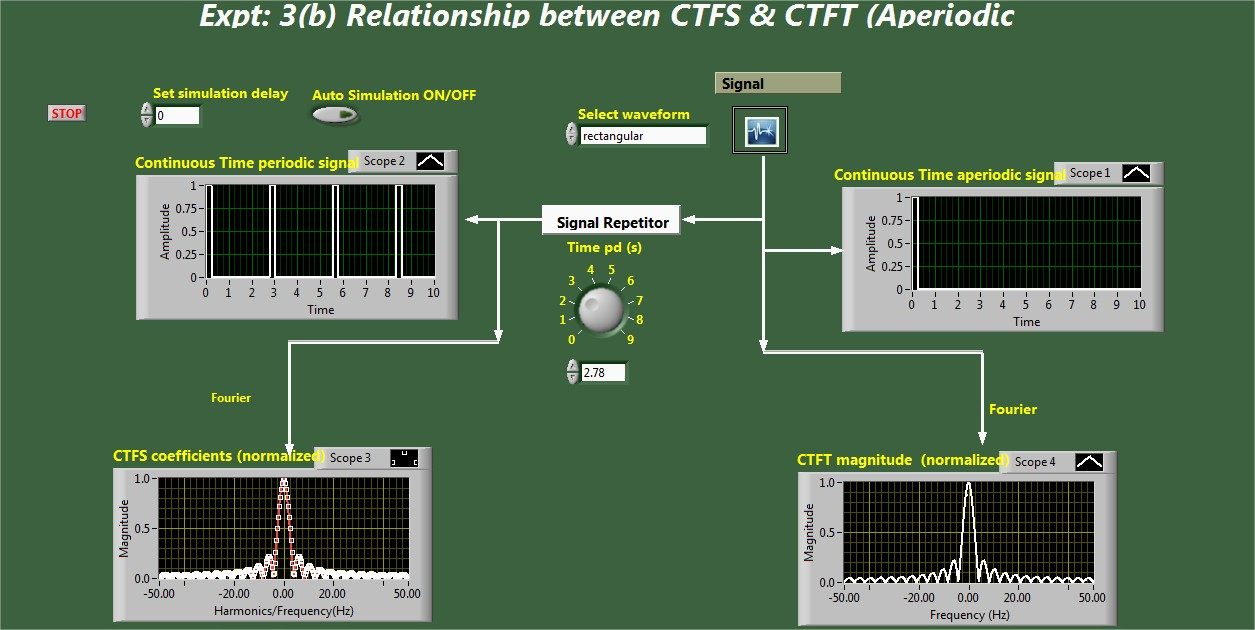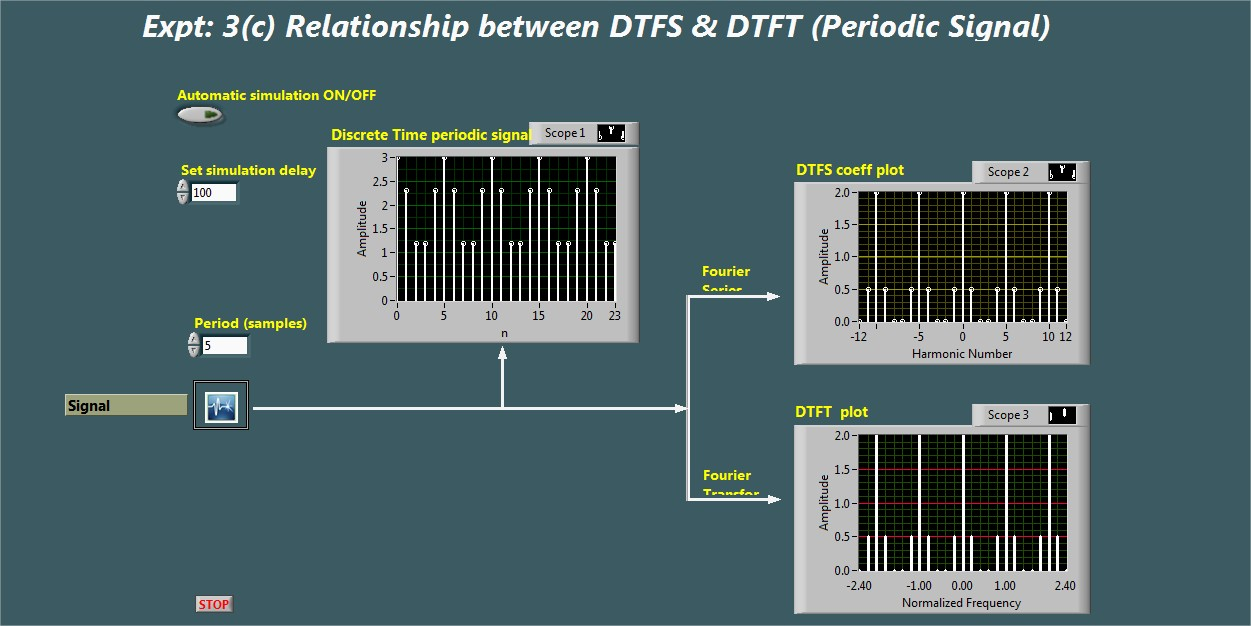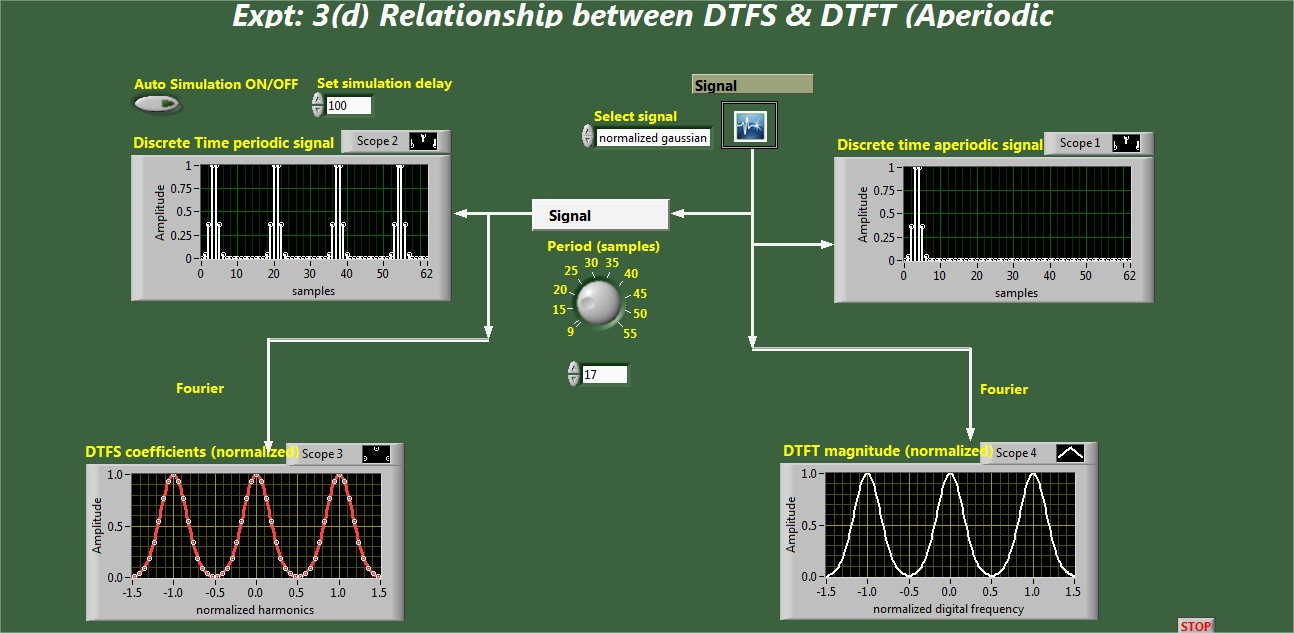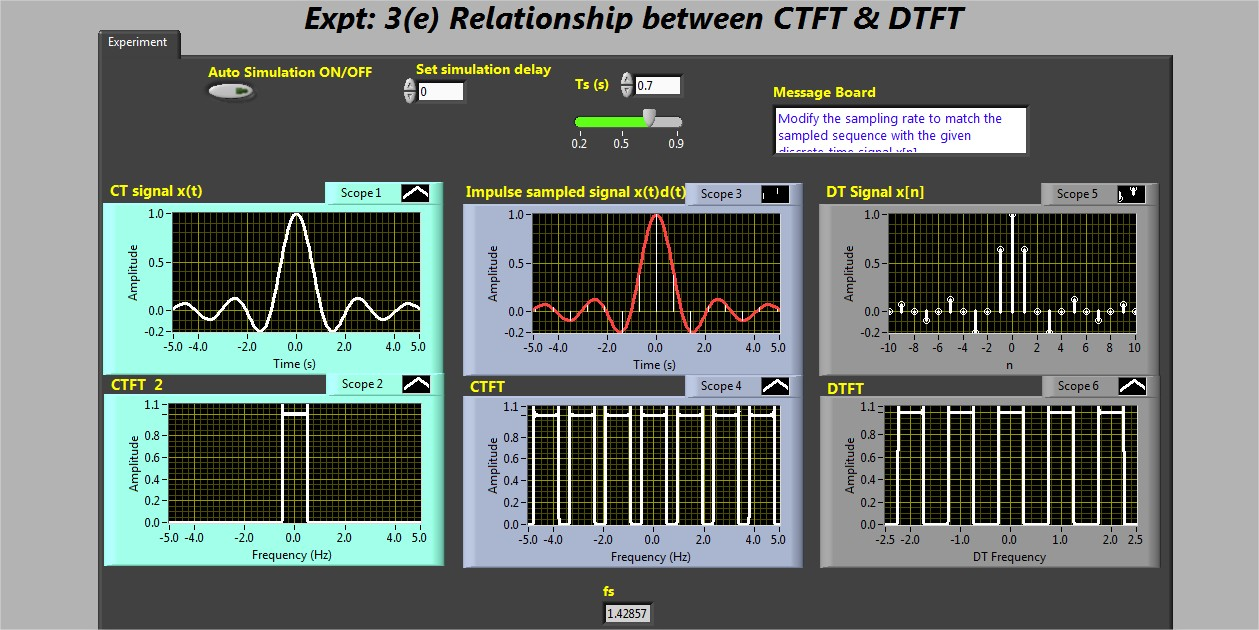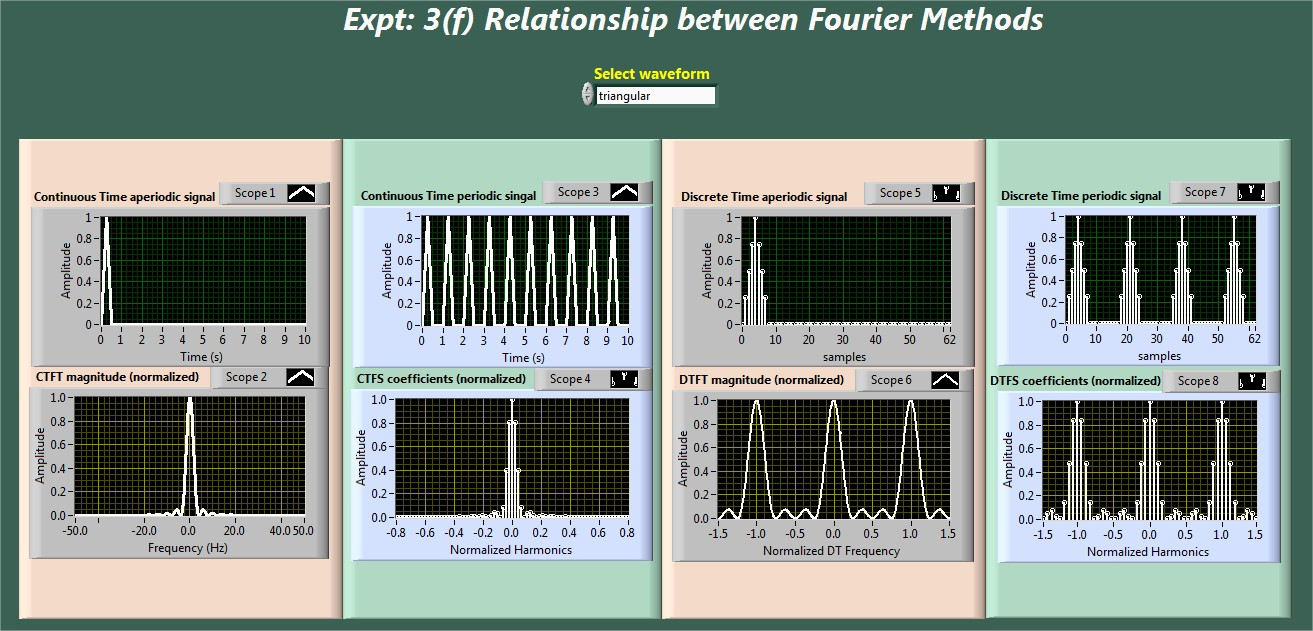# Objectives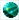To visualize the relationship between the continuous-time Fourier series and Fourier transform of a signal.To visualize the relationship between the discrete-time Fourier series and Fourier transform of a signal.To visualize the relationship between continuous-time and discrete-time Fourier transform of a signals.To visualize the relationship among Fourier analysis methods.

Snapshot of the experiment windows